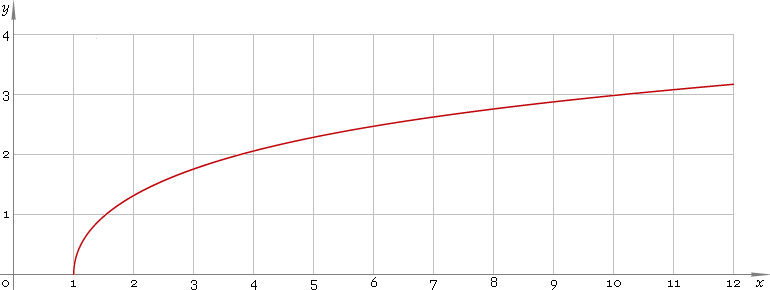The Art of Interface

# arcosh or arch — arc-hyperbolic cosine function

Category. Mathematics.

Abstract. Arc-hyperbolic cosine: definition, plot, properties and identities.

## 1. Definition

Arc-hyperbolic cosine is inverse of hyperbolic cosine function. With the help of natural logarithm it can be represented as:

arcoshx ≡ ln[x + √(x2 − 1)]

## 2. Plot

Arc-hyperbolic cosine is monotone function defined in the range [1, +∞). Its plot is depicted below — fig. 1.Fig. 1. Plot of the arc-hyperbolic cosine function y = arcoshx.

Function codomain is non-negative part of real axis: [0, +∞).

## 3. Identities

Reciprocal argument:

arcosh(1/x) = arsechx

Sum and difference:

arcoshx + arcoshy = arcosh{xy + √[(x2 − 1)(y2 − 1)]}
arcoshx − arcoshy = arcosh{xy − √[(x2 − 1)(y2 − 1)]}
arsinhx + arcoshy = arsinh{xy + √[(x2 + 1)(y2 − 1)]} = arcosh[y√(x2 + 1) + x√(y2 − 1)]

## 4. Support

Arc-hyperbolic cosine function arcosh or arch of the real argument is supported by free version of the Librow calculator.

Arc-hyperbolic cosine function arcosh or arch of the complex argument is supported by professional version of the Librow calculator.

## 5. How to use

To calculate arc-hyperbolic cosine of the number:

``arcosh(2);``

To calculate arc-hyperbolic cosine of the current result:

``arcosh(rslt);``

To calculate arc-hyperbolic cosine of the number x in memory:

``arcosh(mem[x]);``